### Home > CAAC > Chapter 10 > Lesson 10.1.2 > Problem10-22

10-22.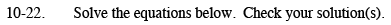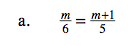Multiply both sides of the equation by a common multiple of 5 and 6.

$30\left(\frac{m}{6}=\frac{m+1}{5}\right)$

5m = 6(m + 1)

Solve for m.
5m = 6m + 6
m = 6

m = −6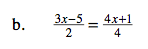Follow the steps in part (a).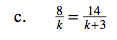A common multiple of k and k + 3 is k(k + 3).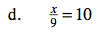Isolate x on one side by undoing the division.

x = 90